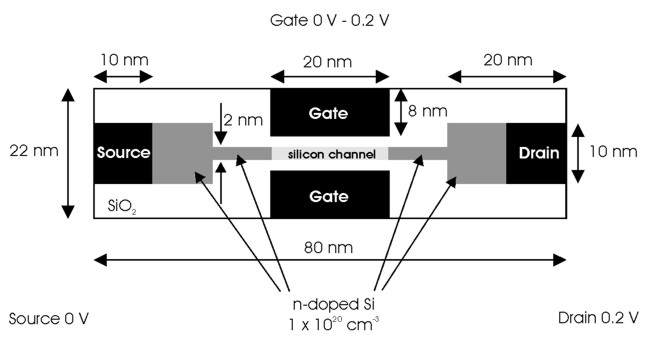nextnano.com  GUI: nextnanomat  Tool: nextnano++  Tool: nextnano³  Tool: nextnano.NEGF  Download | Search | Copyright | News | Publications  * password protected nextnano³ softwareVoltage sweep

# Voltage sweep

Command to perform several calculations with increasing (decreasing) bias voltage at a specific contact (`poisson-cluster-number` / Poisson boundary condition). Useful to get I-V characteristics (current-voltage characteristics) or to get better convergence for high bias voltage by stepwise approaching the final voltage. Sweep always starts from voltage set in Poisson cluster definition (`\$poisson-boundary-conditions`).

With the specifier `'sweep-number'```` up to four sweeps maybe executed simultaneously. Note that the numbers ``` `'sweep-number'```` have to be in consecutive order. The relation between the sweep index appended to each output file ("````_ind000````") and the actual sweep voltage applied is given in the file "sweep_index_to_voltage.dat".```
Please have a look at the voltage sweep tutorial.

```!------------------------------------------------------------! \$voltage-sweep                                     optional  !  sweep-number                       integer        required  !  sweep-active                       character      required  !  poisson-cluster-number             integer_array  required  !  number-of-steps                    integer        required  !  step-size                          double_array   optional  !  table-of-voltages                  double_array   optional  !  data-out-every-nth-step            integer        optional  ! \$end_voltage-sweep                                 optional  ! !------------------------------------------------------------!```

Important:

The output files that are generated during a voltage sweep include an index number in the file name that specifies the step number to which the file belongs. Since

Example:` cb_band001_ind000.dat` : This is the first conduction band output before the first voltage step occurred.
`        cb_band001_ind001.dat` : This is the first conduction band output after the first voltage step occurred.
`        ...`
`        cb_band001_ind00n.dat` : This is the first conduction band output after the nth voltage step occurred.

Examples

```\$voltage-sweep  sweep-number            = 1  sweep-active            = yes  poisson-cluster-number  = 2  step-size               = 0.05d0    ! [V]  number-of-steps         = 10  data-out-every-nth-step = 1 \$end_voltage-sweep```

```\$voltage-sweep  sweep-number            = 1  sweep-active            = yes  poisson-cluster-number  = 1  step-size               = 0.05d0    ! [V]  number-of-steps         = 10  data-out-every-nth-step = 2```

``` sweep-number            = 2  sweep-active            = no  poisson-cluster-number  = 2  step-size               = 0.1d0     ! [V]  number-of-steps         = 3  data-out-every-nth-step = 1 \$end_voltage-sweep```

## Syntax:

```sweep-number            = 1```

`     `Numbering of sweeps. For now the maximum is` 4`. Numbering has to be in consecutive order.

```sweep-active            = yes                         = no```

Flag whether voltage sweep is active or not.

```poisson-cluster-number  = 1                         = 2                         = ...                         = 5                         = 1 3  ! ```Here: Voltage sweep applies to several Poisson clusters simultaneously (Sweep Poisson cluster number `#1 #2`).
```                                !     ``` This might be useful for a 2D or 3D simulation if one has different Schottky barrier heights where the same voltage should be applied or ...

Number of Poisson cluster to which bias voltage should be applied (integer array). The Poisson cluster (= contacts) can be specified here:``` \$poisson-boundary-conditions```

```number-of-steps         = 10```

Number of steps to be performed for this cluster / these clusters.

```step-size               = 0.02d0              ! [V]```

Step size for voltage sweep in [V], should not be larger than 0.1 V.
If more than one` poisson-cluster-number `entries are specified, this entry can consist of an array of different step sizes for each` poisson-cluster-number ` entry.
```                   = 0.02d0  0.01d0      ! [V] ``` Sweep Poisson cluster number `#1 #2 `(``` poisson-cluster-number = 1 3   ! ```(for instance)  )

```                      !   #1         #2        #3       ! ```Sweep Poisson cluster number (``` poisson-cluster-number = 1 4 5 ! ```(for instance)  )``` table-of-voltages       = 0.005d0    0.005d0   0.000d0  ! [V] ```voltages of step 1
```                          0.010d0    0.005d0   0.000d0  ! [V] ```voltages of step 2
```                          0.015d0    0.005d0   0.000d0  ! [V] ```voltages of step 3
```                          0.020d0    0.010d0   0.000d0  ! [V] ```voltages of step 4
```                          0.020d0    0.015d0   0.000d0  ! [V] ```voltages of step 5
```                          0.020d0    0.020d0   0.000d0  ! [V] ```voltages of step 6
```                          0.020d0    0.020d0   1.000d0  ! [V] ```voltages of step 7
```                          0.020d0    0.020d0   2.000d0  ! [V] ```voltages of step 8

Instead of specifying the` step-size`, one can also specify a table of voltages.

The number of entries in` table-of-voltages `must be consistent to the` number-of-steps `and the number of entries in` poisson-cluster-number`.
It must hold:` SIZE(table-of-voltages) = number-of-steps * SIZE(poisson-cluster-number)`

Either` step-size `or` table-of-voltages `must be present but not both of them.

`data-out-every-nth-step = 1`

If you don't want to write out all data specified in the output section for every step, you have to enter an integer number greater than one.
If you specify` 1`, then output files will be generated for each gate voltage. This is useful if you want to fill out all unused space on your hard disk.

### 2D Example with Source, Drain and Gate as contacts (Poisson clusters):`\$voltage-sweep` ``` sweep-active           = yes``` ``` poisson-cluster-number=1``` ``` poisson-cluster-number = 2``` ``` poisson-cluster-number=3``` ``` step-size              = 0.1d0``` ``` number-of-steps        = 4``` sweep-step=0 Source 0.0 V Drain 0.0 V Gate 0.2 V sweep-step=1 Source 0.0 V Drain 0.1 V Gate 0.2 V sweep-step=2 Source 0.0 V Drain 0.2 V Gate 0.2 V sweep-step=3 Source 0.0 V Drain 0.3 V Gate 0.2 V sweep-step=4 Source 0.0 V Drain 0.4 V Gate 0.2 V

For each sweep-step, the Poisson, Schrödinger and current equations are solved for the given voltages. For sweep-step=n, the results of sweep-step=n-1 are taken as starting values to improve convergence.